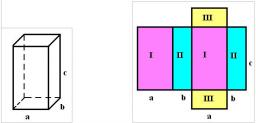# Calculate 6207

The cuboid with a base measuring 17 cm and 13 cm has a surface of 1342 cm2. Calculate the height of the cuboid and sketch its network.

c =  15 cm

### Step-by-step explanation:

1342 = 2·(17·13 + 13·c + c·17)

60c = 900

c = 900/60 = 15

c = 15

Our simple equation calculator calculates it.Did you find an error or inaccuracy? Feel free to write us. Thank you!

Tips for related online calculators
Do you have a linear equation or system of equations and looking for its solution? Or do you have a quadratic equation?